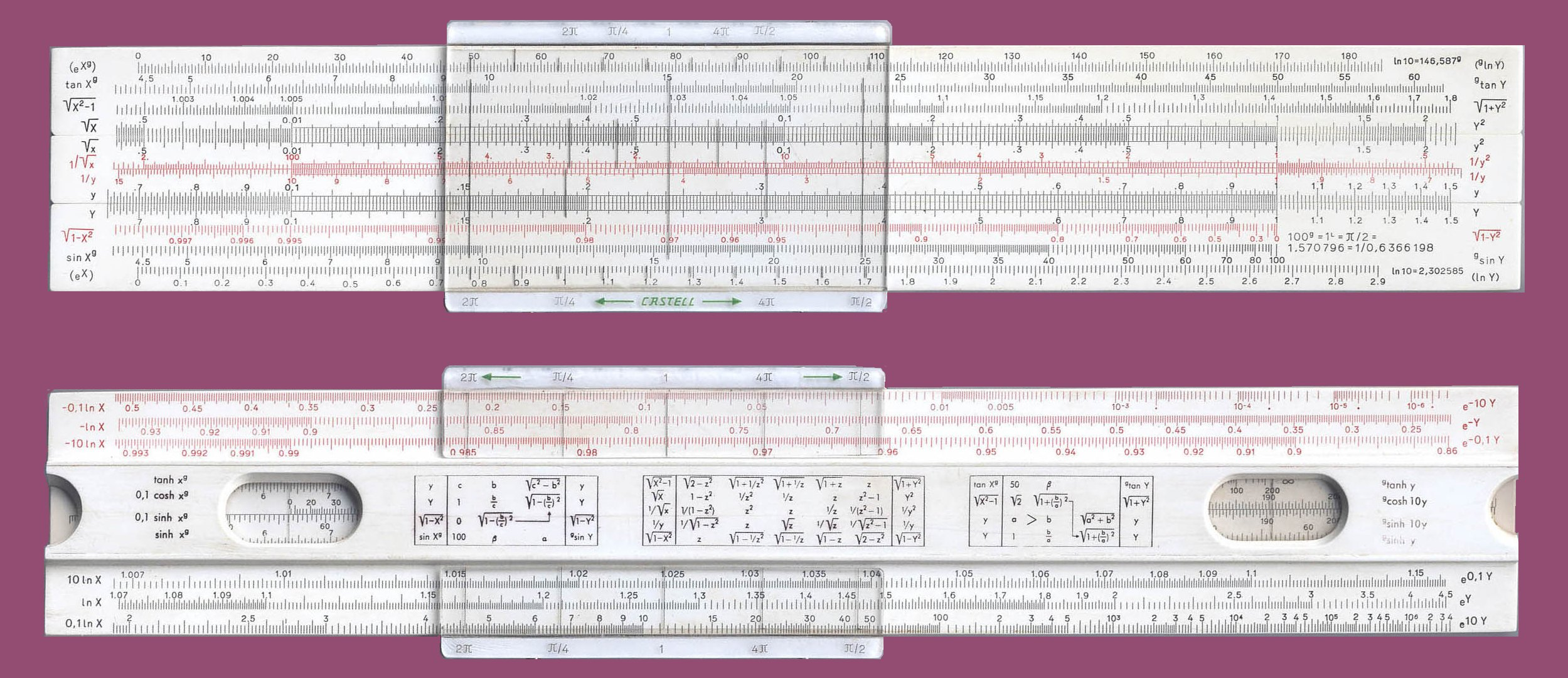Hyperbolic Match Output

Number:27

Maker:FABER-CASTELL (Germany)

Model:Mathema No. 2/84

PHOTO

Scales Front: Left Front: (eXg),tan xg,sqrt(X2-1),sqrt X, || sqrt X, (1 / sqrt X), 1/y, y || Y, sqrt( 1 - X2), sin Xg, (eX) ||
Right Front: || gln Y, gtanY, sqrt(1 + Y2), Y2 || y2, 1/y2, 1/y, y || Y, sqrt(1 - Y2), gsinY, (lnY) ||

Scales Back: Left Back: || -0.1 lnX, -lnX, -10 ln X || tanh xg, 0.1 cosh xg, 0.1 sinh xg, sinh xg || 10 ln X, lnX, 0.1 lnX ||
Right Back: || e-10Y, e-Y, e-0.1Y || gtanh y, gcosh 10y, gsinh 10y, gsinh y || e0.1Y , eY, e10Y ||

Gauge Marks:

Cursor Front: Under the upper two sqrt X scales are two lines to the left at 2π and π/4. To the right are two lines at 4π and π/2. These symbols were printed above on the cursor.

Cursor Back: Under both the the upper and lower scales are two lines at left at 2π and π/4. To the right are two marks at 4π and π/2. These symbols were printed above and below on cursor.

Construction: 20 cm.; Plastic, Cursor is not framed. Closed frame without brackets.

Date: 1966

Source: Rod Lovett Collection

Information: NOTE: The F-C Mathema rules do not use letters to name the scales such as, A, C, CIF, S, etc. Instead formulas or symbols are used to name the scales. NOTE: This is based on 400g system. Where, 400g = 2&pi. Then, 100= π/2, and 1g= π/200. 1 Rad = 200g/π = 63.66198g. π = usual value = 3.1415927